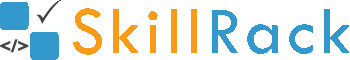ProgramID- 11229SkillRack
Sum of Digits in a Number

The program must accept an integer N as the input. The program must print the sum of all the digits in N as the output.

Boundary Condition(s):
1 <= N <= 10^8

Input Format:
The first line contains N.

Output Format:
The first line contains the sum of all the digits in N.

Example Input/Output 1:
Input:
12345

Output:
15

Explanation:
The sum of all the digits in 12345 is 15.
Hence the output is 15

Example Input/Output 2:
Input:
5000

Output:
5

Max Execution Time Limit: 500 millisecs